# Everyday maths - 7(17^2)

hmmm, its a format of date tho… not a unit of time…

but damn good point

1,1,2,3,5,8,13,21,34,65,99,…,n

the next number would be 164

2 Likes

GIVE ME YOUR BEST MATH PICK UP LINES

uwu nya~

My turnGood luck.

Consider two vectors a,b in the xy plane. The lengths of a and b satisfy the following conditions:

|a| = sqrt(1-x)
|b| = sqrt(2x+1)

for some real number x in [0,1).

Furthermore, the angles between vectors a and b is 30 degrees. (Or pi/6 radians if you’re weird)
(See figure)

Then, the largest value of |a+b| can be expressed in the form of sqrt(x+y*sqrt(z)) / 2 for some positive integers x,y and a positive integer z such that sqrt(z) cannot be simplified further. Find (x,y,z).

Bonus points if you find a method that does not use calculus.

I’ll edit in my solution after… idk when I feel like it.

Just realized I used the variable x twice… oh well they’re different x’s in case you get confused.

me here doing trigo and confused af of what this isPlease put some questions that the younger people can also solve.1 Like

Aight it’s been 16 days and still no answers so I’m just gonna post my solution here.

Solution

Remark:
Considering there were questions about complex numbers and determinants of 3x3 matrices, I just assumed we’re talking about math questions that are approximately high school level math, in which case I don’t believe this question is inappropriate.
Law of cosines is taught in trig class.
Cauchy-Schwarz inequality (n=2) and AM-GM inequality are taught as well.
The numbers may be ugly, but that’s irrelevant.
It is complicated, but it does not involve college math.

p.s. There is a typo in the middle line
Just in case, we need to verify that the value of x which results in this maximum value is in [0,1)
but I can’t be bothered to fix it in the picture so deal with it c:

Cough just busy, I am back now

Cod has many excuses, such as this post was locked…

Anyway it is a new fresh month, let’s start and continue!
Of course I didn’t prepare cough
Question for 60th day in 2023 (1st March):
For three straight lines A, B and C, what is the way to know if they can form a triangle?

the archiving was an accident and i undid it then ._.

Draw all 3 lines on a piece of paper and line them up in a way that’d make a triangle, if they don’t make a triangle they can’t make a triangle.

1 Like

yay a fresh new month!
Cod promises, no, will just try, to continue posting this every day, and includes questions for those days without posts.

Happy April Fool!
Day 91:
Calculate the divorce rate in MythicMC. I don’t have the data so I can’t tell the answer, but if any staff would like to share it, I appreciate for it.

Day 92: 2nd April 2023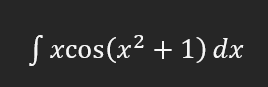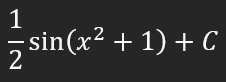Day 93: 3rd April 2023
Question: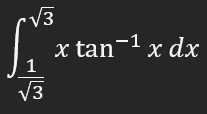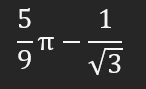1 Like

Day 94: 4th April 2023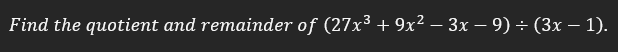1 Like

Try graphing this:

Great graph that represents a fire haircut, ask your barber next time you go to the barbershop to give you one that satisfies the equationi can just call their names lol

Just saw this, I will try to mix.
Also the reason I dislike using forums, is because I don’t want to face this thread lol

Day 174: 23rd June 2023
Area of sector with radius = 7 cm, and angle subtended at the centre is 30 degree.

Answer: Area of the sector ≈ 12.83cm²